##### Signals and Systems For Dummies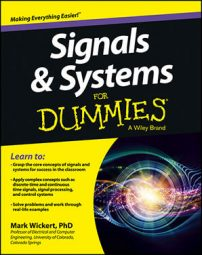The various blocks of a digital communication system are a hybrid of discrete-time signal generation and filtering, continuous-time signal processing at baseband frequencies, and continuous-time radio frequency (RF) up and down conversion.

A digital communications signal at baseband takes the form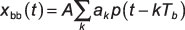where ak is a bit sequence that’s been translated from binary 0/1 values to +/– 1 values, p(t) is a pulse shape, and A is an amplitude scale factor. The bit duration is Th, so the serial bit rate is Rb = 1 / Tb bps. The index k runs over the duration of the digital message sequence.

Common pulse shapes include rectangular (RECT), raised cosine (RC), and square root raised cosine (SRC). In the continuous-time domain, the RECT pulse is just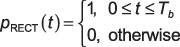In the business of line codes for digital signaling, xbb(t) under the RECT pulse is known as nonreturn-to-zero (NRZ) because the waveform never sits at zero. It’s also common practice to generate xbb(t) first in the discrete-time domain, using the equivalent pulse shape sequence p[n] to create xbb[n] and then using a digital-to-analog converter (DAC) to convert the sequence to a continuous time signal.

For wireless communications, you need to place the baseband signal on a carrier frequency, similar to AM. Because cosine and sine function carriers are orthogonal (in a vector sense, the information carrier by the sine and cosine carriers are at right angles, so they don’t interfere with each other), the typical formulation is to put one baseband signal on cos(2πfct) and a second signal on sin(2πfct).

The result is in-phase quadrature modulation centered at fc: xc(t) = xI(t)cos(2πfct) – xQ(t)sin(2πfct), where xI(t) and xO(t), the in-phase and quadrature signals respectively, are baseband digital data waveforms, like xbb(t).

Binary phase-shift keying (BPSK) is used, which has xI(t) = xbb(t) with RECT, RC, or SRC pulse shaping, and xQ(t) = 0. The block diagram, shown later, is developed for the general case. The name BPSK comes about because with NRZ line coding, xI(t)cos(2πfct) is the carrier signal at either 0 degrees or 180 degrees as a result of the +/– 1 data multiplication.

## Check out the waveform and spectra

Using the custom function NRZ_bits(), written in Python (see ssd.py), the figure shows xI(t) (a discrete-time simulation) and the power spectrum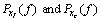```In [<b>547</b>]: x,b,data = ssd.NRZ_bits(100000,50,'rect')
# generate 100000 bits at Ns = 50 samps/bit
In [<b>548</b>]: n = arange(0,len(x))
In [<b>549</b>]: t = n/50. # Rb = 1 bps
In [<b>551</b>]: plot(t,x)
In [<b>553</b>]: psd(x,2**10,50);
In [<b>554</b>]: xc = x*cos(2*pi*12.5*t) # fc = 12.5 Hz
In [<b>556</b>]: psd(xc,2**10,50);```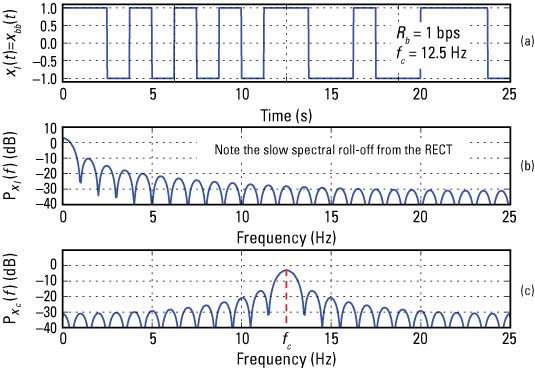Credit: Illustration by Mark Wickert, PhD

The slow spectral roll-off when using the RECT pulse results in poor spectral efficiency. You can resolve this with the RC and SRC pulse shapes.

## Use pulse shapes to increase spectral efficiency

To limit the bandwidth of the RECT spectrum, you can pass the signal through a low-pass filter (such as a low-pass Butterworth, Chebyshev, and so on). Doing so results in intersymbol interference (ISI), which means that signal energy from adjacent bits smear into the bit of interest, raising the probability of creating a bit error when the signal is received in a noise background. So what do you do?

Nyquist pulse shaping to the rescue! The RC pulse shape ensures that no ISI occurs. The bit energy still spreads across multiple symbols, but there’s a timing location once per bit (symbol) period that has zero ISI!

The occupied spectral bandwidth is given by (1 + α)Rb, where 0 < α ≤ 1 is the excess bandwidth factor. Note α → 0 gives the most compact spectrum but is impractical to implement. Typical α values found in use today range from 0.25 to 0.5. The spectral efficiency for BPSK with α = 0.5 is 1.5 bps/Hz.

You must consider power efficiency in digital communications. The idea is to get by, using as little power as possible while maintaining a low probability of making bit errors. Additive noise and interference from other users is the primary cause of bit errors. Additive noise comes about because the received signal strength is usually just above the noise floor (power efficiency).

A matched filter at the receiver ensures that you can reduce the noise yet not overly distort the signal. Nyquist to the rescue again: You can minimize the probability of making a bit error if you distribute the RC pulse shape between the transmitter and receiver matched filter.

You can do this by using the SRC filter. The transmitter pulse shape is SRC, and the receiver matched filter is identical. The SRC pulse has the same parameter α for controlling the signal bandwidth.

Discrete-time implementation of the SRC pulse shapes is the way to go. The mathematical description of the SRC pulse is as follows: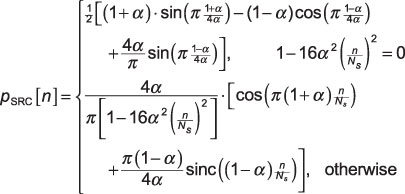Ns is the number of samples per bit (symbol), sinc(x) = sin(πx) / (πx), and 0 < α ≤ 1 is known as the excess bandwidth factor.

A small but significant detail is that the SRC pulse has infinite duration. It’s also symmetrical about n = 0.

## Complete the block diagram

Check out the complete transceiver block diagram for implementing pulse-shaped BPSK as well as general IQ modulation.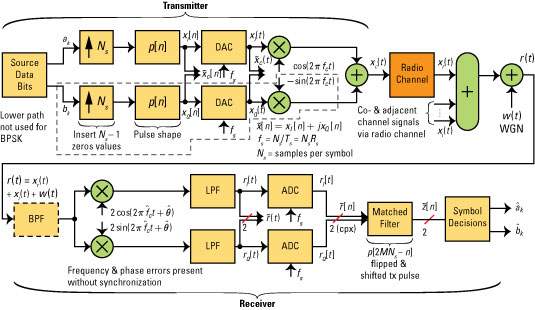Credit: Illustration by Mark Wickert, PhD